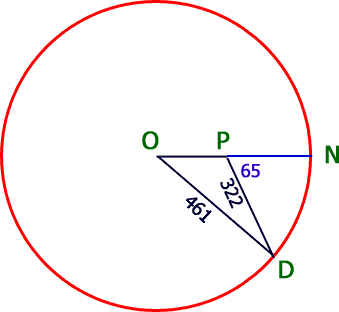SEARCH HOMEMath Central Quandaries & QueriesI was confused on this problem. If a ship leaves port and travel due east. At a certain point it turns 65 degrees south of east and travels b=322 nautical miles to a point 461 nautical miles on a direct line from port. How far from the port is the point where the ship turned?Hi Chris,

I tried drawing the problem. Since the ship ends 461 nautical miles from the origin, we can draw a circle of radius 461 around the origin. At some point P, it pivots 65 degrees southward on its course and proceeds for a distance of 322 nautical miles. Here's the diagram:From the geometry, you can see that angle OPD must be 180 - 65 = 115 degrees. Now you have one angle and two sides of the triangle OPD, so you can use the Law of Cosines to solve for the missing side.

Hope this helps,
Stephen La Rocque.Math Central is supported by the University of Regina and The Pacific Institute for the Mathematical Sciences.